﻿ Mesh zwischen vier geplotteten 3D-Linien - Mein MATLAB Forum - goMatlab.de# Mein MATLAB Forum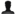Gast > Registrieren       Autologin?
﻿

 Studierende:

 Partner: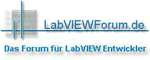Forum
 Option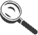Gehe zu: Forum auswählen Allgemeines----------------News zum ForumPro und Contra goMatlab-ForumMathworks News MATLAB - Allgemeine Fragen----------------Grafiken und PlotsGUIsProgrammierung"Suche Funktion für ..." FehlersuchePerformance-SteigerungObjektorientierte ProgrammierungToolboxenMatlab internRelease-FragenSonstige MATLAB FragenBugs MATLAB Simulink / Stateflow / Simscape----------------SimulinkStateflowSimscapeSimMechanics / SimDriveline / SimHydraulics / SimRF / SimElectronics / SimPowerSystemsBugs Simulink / Stateflow / Simscape MATLAB / Simulink / Stateflow - Spezielle Anwendungsgebiete----------------BildverarbeitungMathematikRegelungstechnikSignalverarbeitungCARNOT-Toolboxsonstige Anwendungsgebiete FAQ - MATLAB / Simulink / Stateflow----------------FAQFAQ Diskussion Scripte & Tutorials----------------Script-EckeTutorialsVideo-Tutorials Matlab-Alternativen----------------Scilab / Xcos (ehemals Scicos) ForumOctave-ForumFreeMat-Forum Sonstiges----------------Blog - Mein ProjektProgrammieraufträgeOff Topic Nutzertreffen----------------Allgemeine Diskussion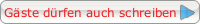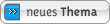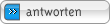## Mesh zwischen vier geplotteten 3D-Linien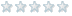Forum-NewbieBeiträge: 5Anmeldedatum: 29.02.08Wohnort: ---Version: ---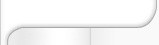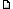Verfasst am: 29.02.2008, 13:40     Titel: Mesh zwischen vier geplotteten 3D-Linien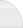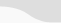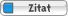Der folgende Matlabcode plottet mir die angehangene Grafik.

 Code: load C:\Ausw7\a.dat; p20 = a(1:2,1); t20 = a(1:2,2); x20 = a(1:2,3); y20 = a(1:2,4); q20 = a(1:2,5); p40 = a(3:5,1); t40 = a(3:5,2); x40 = a(3:5,3); y40 = a(3:5,4); q40 = a(3:5,5); p60 = a(6:8,1); t60 = a(6:8,2); x60 = a(6:8,3); y60 = a(6:8,4); q60 = a(6:8,5); p80 = a(9:11,1); t80 = a(9:11,2); x80 = a(9:11,3); y80 = a(9:11,4); q80 = a(9:11,5); plot3(p20,t20,x20,p40,t40,x40,p60,t60,x60,p80,t80,x80); grid on   Funktion ohne Link?

Ich möchte nun diese vier Graphen mit einem Mesh verbinden.

Wie ist dies bitte möglich?

Danke
Martin

3D.jpg
Beschreibung: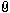Dateiname:  3D.jpg
Dateigröße:  29.66 KB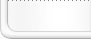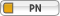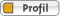ThemenstarterForum-NewbieBeiträge: 5Anmeldedatum: 29.02.08Wohnort: ---Version: ---Verfasst am: 29.02.2008, 16:31     Titel:Mittlerweile habe ich den Code wie folgt geändert.

 Code: load C:\Ausw7\a.dat; p20 = a(1:2,2); t20 = a(1:2,1); x20 = a(1:2,3); y20 = a(1:2,4); q20 = a(1:2,5); p40 = a(3:5,2); t40 = a(3:5,1); x40 = a(3:5,3); y40 = a(3:5,4); q40 = a(3:5,5); p60 = a(6:8,2); t60 = a(6:8,1); x60 = a(6:8,3); y60 = a(6:8,4); q60 = a(6:8,5); p80 = a(9:11,2); t80 = a(9:11,1); x80 = a(9:11,3); y80 = a(9:11,4); q80 = a(9:11,5); p = a(:,2); t = a(:,1); x = a(:,3); y = a(:,4); q = a(:,5); xi = 20:10:80; yi = 0:10:450; [XI,YI] = meshgrid(xi,yi); plot3(t20,p20,x20,t40,p40,x40,t60,p60,x60,t80,p80,x80); hold on ZI = griddata(t,p,x,XI,YI); surf(XI,YI,ZI);   Funktion ohne Link?

Daraufhin bekomme ich folgende Fehlermeldung

 Zitat: ??? Error using ==> qhullmx qhull precision error: initial facet 3 is coplanar with the interior point ERRONEOUS FACET: While executing: | qhull d Qt Qbb Qc Options selected for Qhull 2003.1 2003/12/30: delaunay Qtriangulate Qbbound-last Qcoplanar-keep _pre-merge _zero-centrum Pgood Qinterior-keep _max-width 4.5e+002 Error-roundoff 6.2e-013 _one-merge 4.4e-012 Visible-distance 1.2e-012 U-coplanar-distance 1.2e-012 Width-outside 2.5e-012 _wide-facet 7.5e-012 precision problems (corrected unless 'Q0' or an error) 2 flipped facets The input to qhull appears to be less than 3 dimensional, or a computation has overflowed. Qhull could not construct a clearly convex simplex from points: The center point is coplanar with a facet, or a vertex is coplanar with a neighboring facet. The maximum round off error for computing distances is 6.2e-013. The center point, facets and distances to the center point are as follows: facet p7 p3 p0 distance= -2.8e-014 facet p10 p3 p0 distance= -2.8e-014 facet p10 p7 p0 distance= 0 facet p10 p7 p3 distance= 0 These points either have a maximum or minimum x-coordinate, or they maximize the determinant for k coordinates. Trial points are first selected from points that maximize a coordinate. The min and max coordinates for each dimension are: 0: 20 80 difference= 60 1: 0 450 difference= 450 2: 0 450 difference= 450 If the input should be full dimensional, you have several options that may determine an initial simplex: - use 'QJ' to joggle the input and make it full dimensional - use 'QbB' to scale the points to the unit cube - use 'QR0' to randomly rotate the input for different maximum points - use 'Qs' to search all points for the initial simplex - use 'En' to specify a maximum roundoff error less than 6.2e-013. - trace execution with 'T3' to see the determinant for each point. If the input is lower dimensional: - use 'QJ' to joggle the input and make it full dimensional - use 'Qbk:0Bk:0' to delete coordinate k from the input. You should pick the coordinate with the least range. The hull will have the correct topology. - determine the flat containing the points, rotate the points into a coordinate plane, and delete the other coordinates. - add one or more points to make the input full dimensional. This is a Delaunay triangulation and the input is co-circular or co-spherical: - use 'Qz' to add a point "at infinity" (i.e., above the paraboloid) - or use 'QJ' to joggle the input and avoid co-circular data Error in ==> delaunayn at 114 t = qhullmx(x', 'd ', opt); Error in ==> griddata>linear at 151 tri = delaunayn([x y]); Error in ==> griddata at 120 zi = linear(x,y,z,xi,yi,opt);

Zum Nachvollziehen hier meine Werte aus der Datei a.dat:

20 0 0 0 0
20 450 0.2435 0.7466 0.519
40 0 -0.0066 -0.015 -0.0062
40 200 0.1064 0.3423 0.2257
40 450 0.2621 0.7347 0.5102
60 0 0.0001 -0.0373 -0.0136
60 200 0.1157 0.3084 0.2261
60 450 0.3638 0.7225 0.5100
80 0 0.0063 0.0466 0.0337
80 200 0.1094 0.3037 0.1910
80 450 0.2982 0.7360 0.4591

1) Ich kann diese Fehlermeldung nicht deuten. Was bedeutet diese?
2) Wie bekomme ich den mesh bzw. surf hin?

Tausend Dank
MartinisThemenstarterForum-NewbieBeiträge: 5Anmeldedatum: 29.02.08Wohnort: ---Version: ---Verfasst am: 29.02.2008, 17:12     Titel: Problem erkannt, Gefahr gebanntNach über drei Stunden habe ich das Problem selber lösen können

Die Lösung 'v4':

 Code: ZI = griddata(t,p,x,XI,YI,'v4');   Funktion ohne Link?

Nu kann ich in den Feierabend gehenEinstellungen und Berechtigungen Beiträge der letzten Zeit anzeigen: Alle Beiträge1 Tag7 Tage2 Wochen1 Monat3 Monate6 Monate1 Jahr Die ältesten zuerstDie neusten zuerst Du kannst Beiträge in dieses Forum schreiben.Du kannst auf Beiträge in diesem Forum antworten.Du kannst deine Beiträge in diesem Forum nicht bearbeiten.Du kannst deine Beiträge in diesem Forum nicht löschen.Du kannst an Umfragen in diesem Forum nicht mitmachen.Du kannst Dateien in diesem Forum postenDu kannst Dateien in diesem Forum herunterladen
 .

 goMatlab ist ein Teil des goForen-Labels

Impressum  | Nutzungsbedingungen  | Datenschutz  | Werbung/Mediadaten | Studentenversion | FAQ |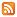RSS

Copyright © 2007 - 2021 goMatlab.de | Dies ist keine offizielle Website der Firma The Mathworks
Partner: LabVIEWforum.de

MATLAB, Simulink, Stateflow, Handle Graphics, Real-Time Workshop, SimBiology, SimHydraulics, SimEvents, and xPC TargetBox are registered trademarks and The MathWorks, the L-shaped membrane logo, and Embedded MATLAB are trademarks of The MathWorks, Inc.